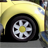# Average value inside repeats

1059
3
01-15-2019 01:55 AMOccasional Contributor

Hi, Is there any option to calculate the average (the sum of each value entered divided by the number of values entered into a repetition (has no defined value) and can be calculated?

p.e.

"If you enter 02 values, then divided by 02, if you enter 03 values, divided by 03, if you enter N values divided by N "

I think I've only seen it within ideas of improvements.

Tags (2)
3 RepliesOccasional Contributor

It can be done. Here is how I do it in my survey:

Create 2 field Outside the  reapeat group. One field will use the function Count() on a field within the repeat group and the other will use the Sum() function on that same field.

Then simply divide the results.Occasional Contributor

Thanks, NicolasNew Contributor II

Alternatively, create a new row (or field) outside the repeat to calculate the average. The calculation would look something like this:

(sum(\${Question_1})) div (count(\${Question_1}))